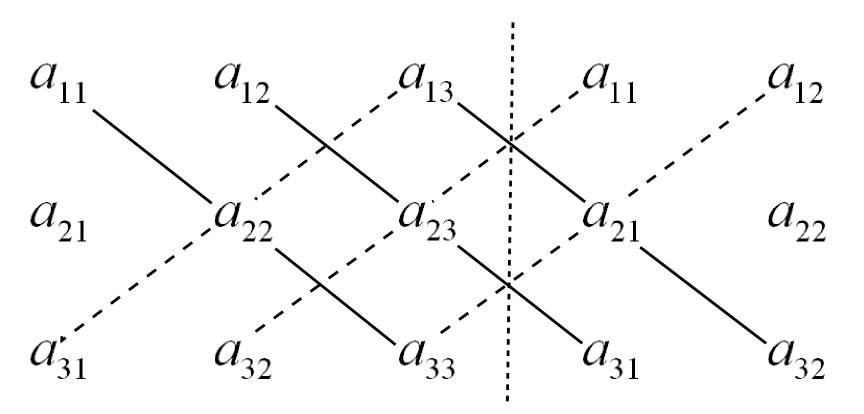My colleague K.-J. Wirths came up with another Rule of Sarrus for${4\times 4}$ matrices. His suggestion is somehow closeto the original (at least graphically) and is easier to memorize. One has to use the “original” Rule of Sarrus for the${4\times 4}$ case but now three times. For the first case use the original matrix and for the next two case one has to permute two columns. Graphically this gives the following the pictures:In principle this generalizes to larger${n\times n}$ matrices. But beware:${n!}$ is large! For the${5\times 5}$ case one has a sum of 120 products but each “standard Sarrus” only gives 10 of them. Hence, one has to figure out 12 different permutations. In the${n\times n}$ case one even needs to memorize${\frac{n!}{2n}}$ permutation, let alone all the computations…

I am sure that somebody with stronger background in algebra and more knowledge about permutation groups could easily figure out what is going on here, and to visualize the determinants better.

Update: Indeed! Somebody with more background in algebra already explored how to generalize the Sarrus rule to larger matrices. Again it was my colleague K.-J. Wirths who found the reference and here it is:

• Обобщенное правило Саррюса, by С. Аршон, Матем. сб., 42:1 (1935), 121–128

and it is from 1935 already! If you don’t speak Russian, in German it is

• “Verallgemeinerte Sarrussche Regel”, S. Arschon, Mat. Sb., 42:1 (1935), 121–128

and if you don’t speak German either, you can visit the page in mathnet.ru or to the page in the Zentralblatt (but it seems that there is no English version of the paper or the abstract available…) Anyway, you need$(n-1)!/2$ permutations of the columns and apply the plain rule of Sarrus to all these (and end up, of course, with$2n(n-1)!/2=n!$ summands, each of which has$n$ factors – way more than using LU of QR factorization.)

There is this famous Rule of Sarrus for calculating the determinant of a${3\times 3}$ matrix. The nice thing is, that one can remember it quite quickly with the help of a simple picture which I cite from the Wikipedia page:Being at a university which has a lot of engineers who need to learn mathematics, there are plenty of students who learn about determinants and also learn the Rule of Sarrus. A quite amusing thing which happens frequently in exams is the following (at least colleagues told me so): If you ask the students to calculate the determinant of a${4\times 4}$ matrix there will be a number of people adopting the Rule of Sarrus without thinking, ending up with the sum of eight products. You will also find interesting discussions if you google “Sarrus 4×4”. Of course, the Rule of Sarrus can not work in this simple manner since, according to the Leibniz formula for determinants, you need a sum of 24 products.

Well, I thought it could be nice to have an illustration of the 24 “paths” along which you should take products in a${4\times 4}$ matrix. My first idea was to you Laplace’s formula for the first column. That is, I use the formula$\displaystyle \det(A) = \sum_{i=1}^4 (-1)^{i+1}a_{i,1}M_{i,1}$

in which${M_{i,1}}$ is the determinant of the${3\times 3}$ sub-matrix obtained by removing the first column and the${i}$th row of${A}$. For the entry${a_{1,1}}$ this gives this picture:Here, the green lines indicate products which get the sign${+}$ and red lines indicate products which get the sign${-}$. The blue dots are just for better orientation.

Similarly, the elements${a_{2,1}}$,${a_{3,1}}$ and${a_{4,1}}$ lead to similar pictures:Putting all graphics together we obtain the nice and intuitive picturefor the 4×4 Rule of Sarrus. Ok – have fun memorizing this…

Since this picture looks so ugly, one may be tempted to call the corresponding rule the “Rule of Sauron”…

P.S.: Probably there is some graph theorist somewhere who could produce a nicer picture, e.g. one which minimizes the number of crossing. Moreover, there are probably other thoughts about the Ruls of Sarrus and its interpretations for larger matrices – I would be glad to learn about them.

In a follow-up post, I show a simpler visualization.